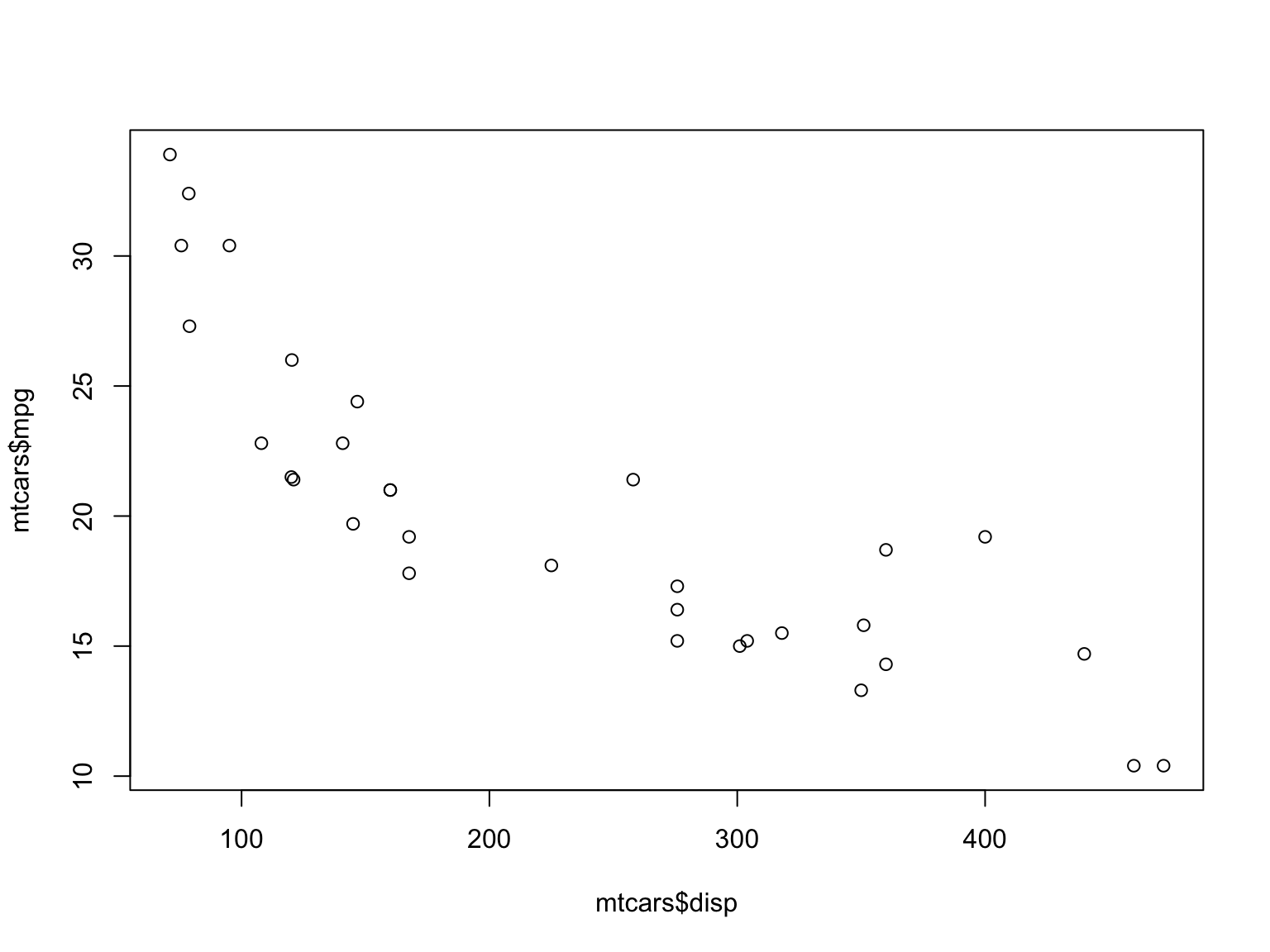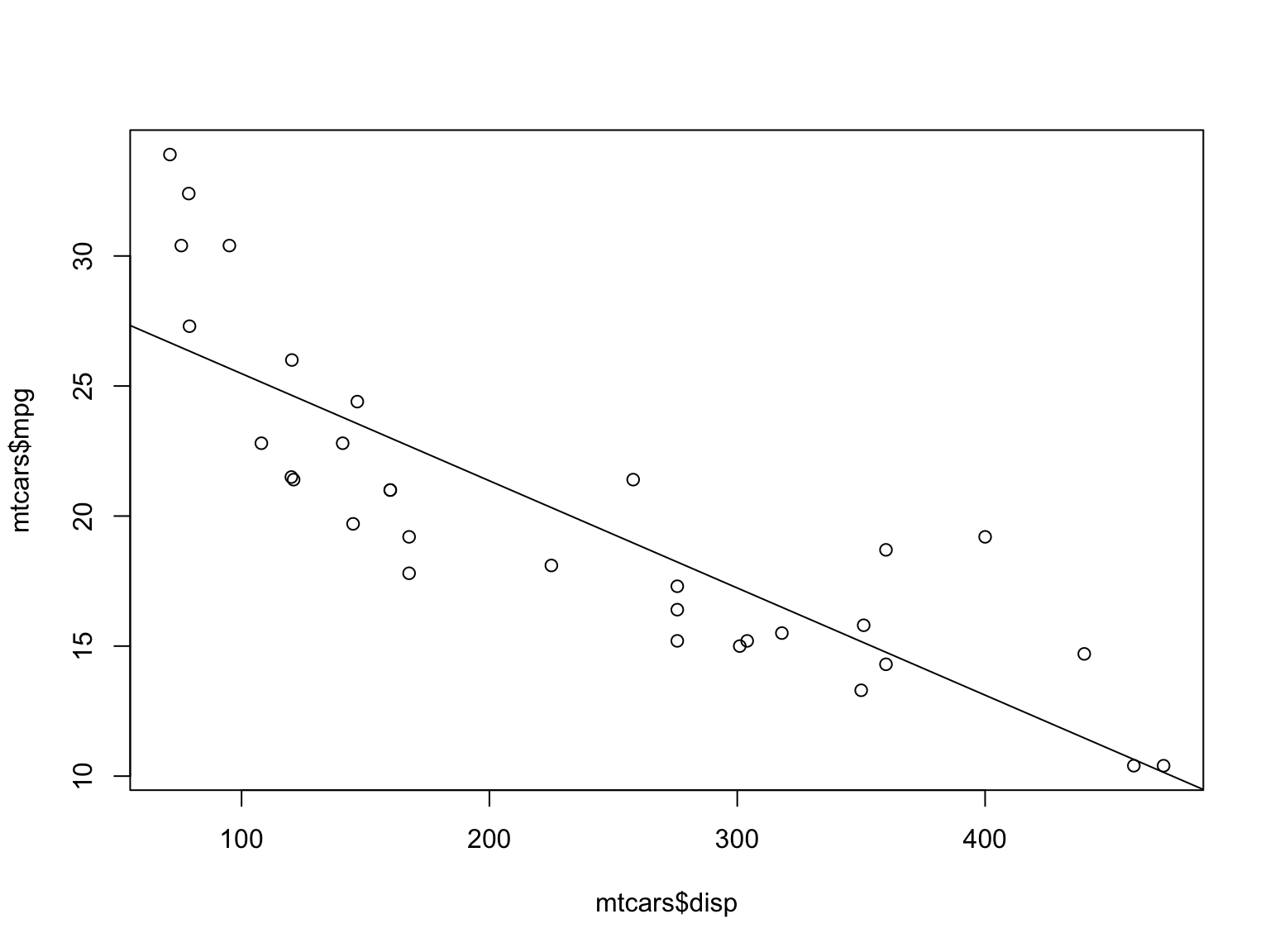April 16, 2016

# A Simple Regression Example

We will use the data in the builtin daaframe mtcars.

First let’s examine the relatioship bwtween the engine displacement (explanatory) and mpg (response) graphically. We expect greater displacement to be associated with reduced mpg. A scatterplot should show that points farther to the right are lower. The correlation coeffieient should be negative and not very close to zero, probably close to -1.

plot(mtcars$disp,mtcars$mpg)cor(mtcars$disp,mtcars$mpg)
##  -0.8475514

We can take this a step further and create a model of the relationship between engine displacement and gas mileage using linear regression.

The idea is to assume that there is a linear relationship of the form

$mpg = m*disp+b$

We can use the function lm() in R to derive estimates of the parameters $$m$$ and $$b$$ from the existing data. You should recognize this as the slope-intercept form of a straight line. The slope, $$m$$ is the more important of these two parameters. It tells us how much gas mileage will change and in which direction when engine displacement increases. We expect it to have a negative value in this case.

lm1 <- lm(mpg~disp,data = mtcars)
summary(lm1)
##
## Call:
## lm(formula = mpg ~ disp, data = mtcars)
##
## Residuals:
##     Min      1Q  Median      3Q     Max
## -4.8922 -2.2022 -0.9631  1.6272  7.2305
##
## Coefficients:
##              Estimate Std. Error t value Pr(>|t|)
## (Intercept) 29.599855   1.229720  24.070  < 2e-16 ***
## disp        -0.041215   0.004712  -8.747 9.38e-10 ***
## ---
## Signif. codes:  0 '***' 0.001 '**' 0.01 '*' 0.05 '.' 0.1 ' ' 1
##
## Residual standard error: 3.251 on 30 degrees of freedom
## Multiple R-squared:  0.7183, Adjusted R-squared:  0.709
## F-statistic: 76.51 on 1 and 30 DF,  p-value: 9.38e-10
plot(mtcars$disp,mtcars$mpg)
abline(lm1)Note that we need to ask for the summary of the model we create since creating a model does not automatically display it.

The value of $$m$$ is given as $$-.041$$ in the output. The meaning of this is that increasing the value of displacement by one unit will decrease mpg by .041 units. This is easier to grasp by thinking of the impact of an extra 100 cubic inches of engine displacement, which would drive a decrease of 4 mpg in gas mileage.

Recreating the scatterplot and adding the regression line to the plot is useful to judge the usefulness of the model.

# Exercise

Repeat the steps above to examine the relationship between the weight of the vehicle and mpg.

# Two Independent Variables

Consider both weight and engine size.

lm3 <- lm(mpg~wt+disp,data=mtcars)
summary(lm3)
##
## Call:
## lm(formula = mpg ~ wt + disp, data = mtcars)
##
## Residuals:
##     Min      1Q  Median      3Q     Max
## -3.4087 -2.3243 -0.7683  1.7721  6.3484
##
## Coefficients:
##             Estimate Std. Error t value Pr(>|t|)
## (Intercept) 34.96055    2.16454  16.151 4.91e-16 ***
## wt          -3.35082    1.16413  -2.878  0.00743 **
## disp        -0.01773    0.00919  -1.929  0.06362 .
## ---
## Signif. codes:  0 '***' 0.001 '**' 0.01 '*' 0.05 '.' 0.1 ' ' 1
##
## Residual standard error: 2.917 on 29 degrees of freedom
## Multiple R-squared:  0.7809, Adjusted R-squared:  0.7658
## F-statistic: 51.69 on 2 and 29 DF,  p-value: 2.744e-10

# Two Independent Variables with Interaction

lm4 <- lm(mpg~wt+disp+disp*wt,data=mtcars)
summary(lm4)
##
## Call:
## lm(formula = mpg ~ wt + disp + disp * wt, data = mtcars)
##
## Residuals:
##    Min     1Q Median     3Q    Max
## -3.267 -1.677 -0.836  1.351  5.017
##
## Coefficients:
##              Estimate Std. Error t value Pr(>|t|)
## (Intercept) 44.081998   3.123063  14.115 2.96e-14 ***
## wt          -6.495680   1.313383  -4.946 3.22e-05 ***
## disp        -0.056358   0.013239  -4.257  0.00021 ***
## wt:disp      0.011705   0.003255   3.596  0.00123 **
## ---
## Signif. codes:  0 '***' 0.001 '**' 0.01 '*' 0.05 '.' 0.1 ' ' 1
##
## Residual standard error: 2.455 on 28 degrees of freedom
## Multiple R-squared:  0.8501, Adjusted R-squared:  0.8341
## F-statistic: 52.95 on 3 and 28 DF,  p-value: 1.158e-11
predict(lm4,data.frame(wt=c(2,3,3),disp=c(250,250,350)))
##        1        2        3
## 22.85381 19.28448 17.16029